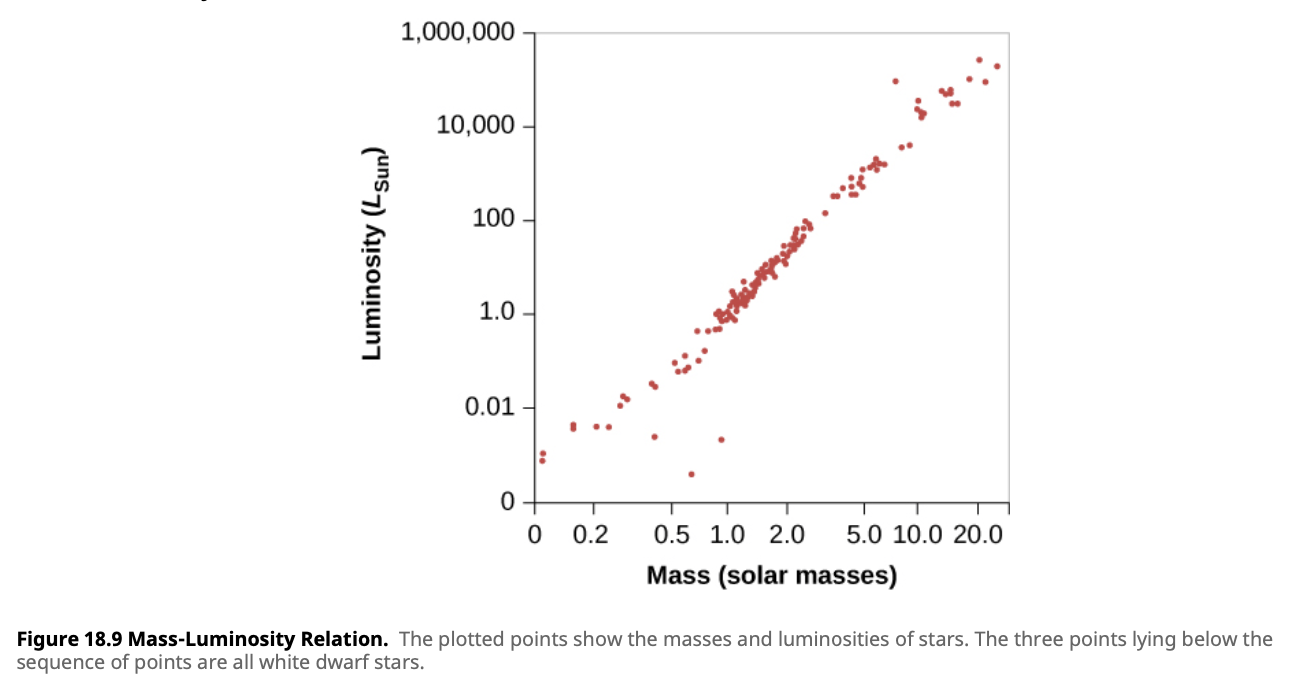# Mass-Luminosity Relation• The more massive stars are generally the more luminous
• Luminosity varies as the fourth power of the mass
• Can be used to find the distance to binary star systems

## Formula

• $\frac{L}{L_{sun}} = (\frac{M}{M_{sun}})^a$